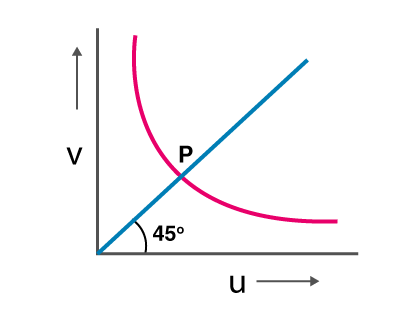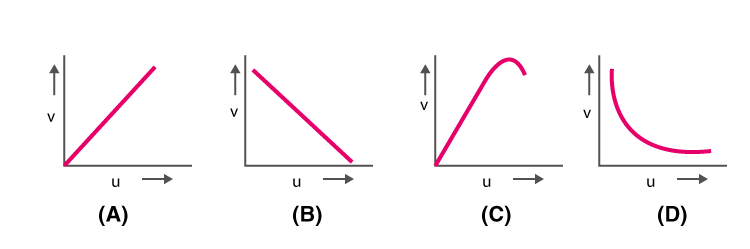# Optics MCQs for NEET

Optics is one of the branches of Physics that deals with the behaviour and properties of light. It is used for describing the behaviour of visible light, infrared, and ultraviolet light. Reflection, refraction, total internal reflection, dispersion, etc are the properties exhibited by the light and these properties are studied in optics.

Geometrical optics, physical optics, and quantum optics are the three different types of optics. The study of light as rays is done in geometrical optics. Physical optics is the study of light as waves while quantum optics is the study of light as particles.

1. Calculate the velocity of the light ray in the medium, if the critical angle for TIR from medium to the vacuum is 30°.

(a) 2 x 108 m/s

(b) 1.5 x 108 m/s

(c) 0.75 x 108 m/s

(d) 3 x 108 m/s

Answer: (b) 1.5 x 108 m/s

2. Which of the following is the combination that is used in the formation of achromatic lenses?

(a) 1 convex and 1 plane mirror

(b) 2 convex lenses

(c) 1 convex and 1 concave lens

(d) 2 concave lenses

Answer: (c) 1 convex and 1 concave lens

3. How does magnifying power change for an objective lens if the focal length of the objective lens is increased?

(a) The microscope will decrease but for the telescope, it will increase

(b) The microscope will increase but for the telescope, it decreases

(c) The microscope and telescope will increase

(d) The microscope and telescope will decrease

Answer: (a) The microscope will decrease but for the telescope, it will increase

4. What is the power of the combination of lenses, if two thin lenses of focal length f1 and f2 are in contact and coaxial.

(a) $$\frac{f_{1}-f_{2}}{f_{1}f_{2}}$$

(b) $$\frac{f_{2}}{f_{1}}$$

(c) $$\sqrt{\frac{f_{1}}{f_{2}}}$$

(d) $$\frac{f_{1}+f_{2}}{f_{1}f_{2}}$$

Answer: (d) $$\frac{f_{1}+f_{2}}{f_{1}f_{2}}$$

5. Following is the graph explaining the variation of v with respect to u for a mirror. What is the value of points above the marking P on the curve for values of v(a) Smaller than f

(b) Larger than 2f

(c) Smaller than 2f

(d) Smaller than 2

6. What is the magnification produced by the plane mirror?

(a) Zero

(b) +1

(c) -1

(d) Between 0 and +∞

7. The image formed by the convex mirror is $$\frac{1}{n}$$ times the object and has a focal length f. What is the distance of object from the mirror?

(a) (n + 1)f

(b) (n – 1)f

(c) $$(\frac{n+1}{n})f$$

(d) $$(\frac{n-1}{n})f$$

8. Which of the following graph is correct when the real image distance v formed by a convex lens is measured for different object distance u.9. What happens to the refractive index on heating the liquid?(a) Increases

(b) Decreases

(c) Remains unchanged

(d) Increases or decreases depending on the rate of heating

10. Calculate the speed of light in water if the refractive index of water is 1.33(a) 4 x 108 m/s

(b) 2.25 x 108 m/s

(c) 1.33 x 108 m/s

(d) 3 x 108 m/s

Answer: (b) 2.25 x 108 m/s

11. What is hypermetropia?

(a) Bad vision due to age

(b) Short-sightedness

(c) Long-sightedness

(d) Formation of a layer on the eye

12. Rainbow is an example of which phenomenon?

(a) Refraction and scattering

(b) Refraction and total internal reflection

(c) Dispersion and reflection

(d) Dispersion and total internal reflection

Answer: (d) Dispersion and total internal reflection